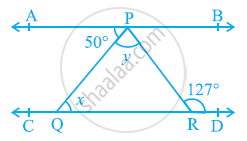# In the given figure, if AB || CD, ∠APQ = 50º and ∠PRD = 127º, find x and y. - Mathematics

#### Question

In the given figure, if AB || CD, ∠APQ = 50º and ∠PRD = 127º, find x and y.#### Solution

∠APR = ∠PRD  (Alternate interior angles)

50º + y = 127º

y = 127º − 50º

y = 77º

Also, ∠APQ = ∠PQR   (Alternate interior angles)

50º = x

∴ x = 50º and y = 77º

Is there an error in this question or solution?

#### APPEARS IN

NCERT Solution for Mathematics Class 9 (2018 (Latest))
Chapter 6: Lines and Angles
Exercise 6.2 | Q 5 | Page 105

#### Video TutorialsVIEW ALL 

In the given figure, if AB || CD, ∠APQ = 50º and ∠PRD = 127º, find x and y. Concept: Lines Parallel to the Same Line.